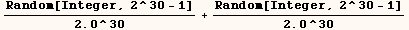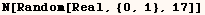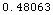Random

On 5 May 2000 Daniel Lichtblau of Wolfram Research reported at
http://forums.wolfram.com/mathgroup/archive/2000/May/msg00088. html
that the numbers produced using to produce rando approximate machine  numbers are not completely random.  Further more at
http://forums.wolfram.com/mathgroup/archive/2004/May/msg00002. html
Andrzej Kozlowski reported that this is still a problem in Mathematica 5.0.
Daniel Lichtblau suggests that instead of using  Random[]  to  generate random real machine numbers the following should be used.  I believe  this will give a random number between 1 and 2.However, based on Daniel's explanation of the problem, I expect that the
following also works around the bug in Random, and runs twice as fast as the
solution above.In the above message Daniel Lichtblau also explained how one can make
necessary changes to some of the standard packages.

Created by Mathematica  (May 16, 2004)

Back to Ted’s Tricks index page